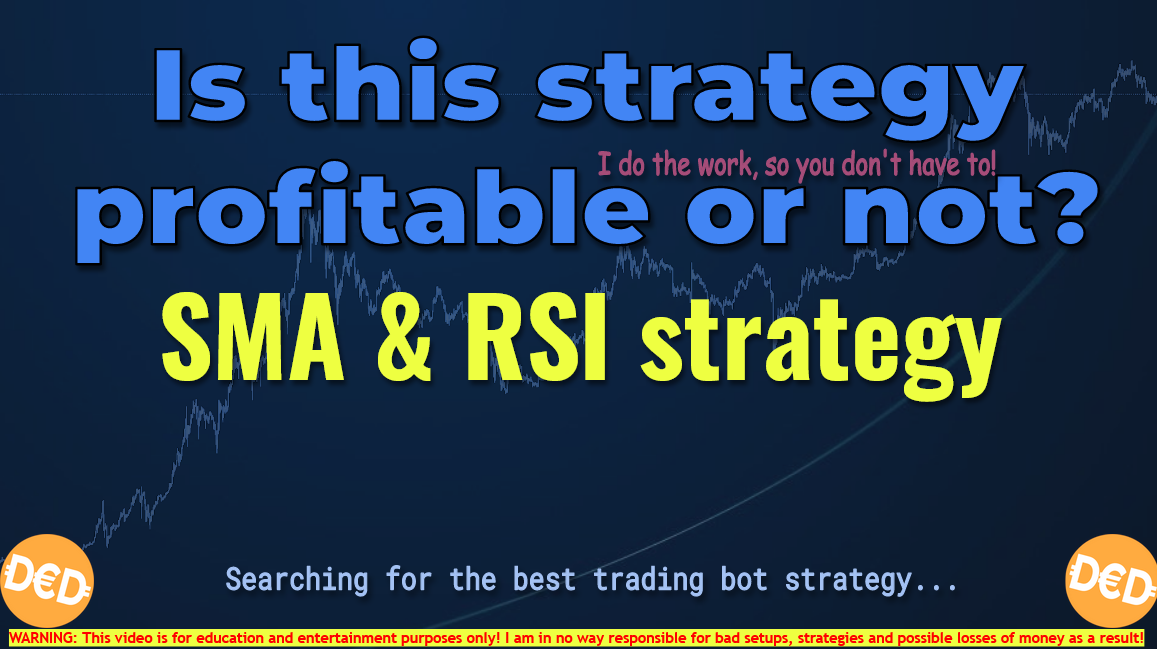# SMA & RSI trading strategy. Is it profitable or not?

### Intro

This is the blog post that complementary to the video where I am going to test the 21 simple moving average in combination with the RSI 14

This is a very simple strategy and I am going to test if this is also good for trading crypto pairs with the help of a trading bot.

### The setup

See this blog post to know more about the approach I use when making these backtests: https://www.publish0x.com/dutchcryptodad/is-it-profitable-or-not-the-setup-and-approach-xppgjoj

### Strategy

The buy and sell settings are very easy to understand.
Buy when the close price is above the simple moving average and when the rsi is above the 50 level

Sell when the price is below the sma and rsi is below the 50 level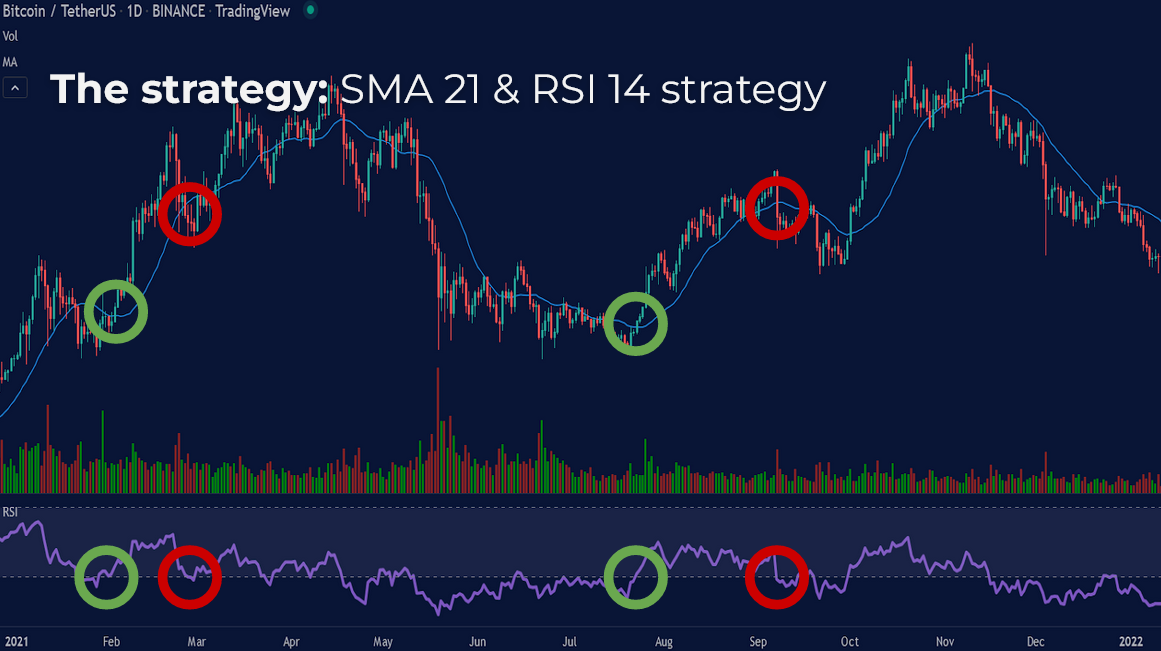### Stoploss and takeprofit

Besides the entry and exit signals of the strategy based on the indicators, there are also stoploss and takeprofit points that can be used.

These stoploss and takeprofit levels are later on determined by the hyperopt functionality of the bot and are percentage based exit levels.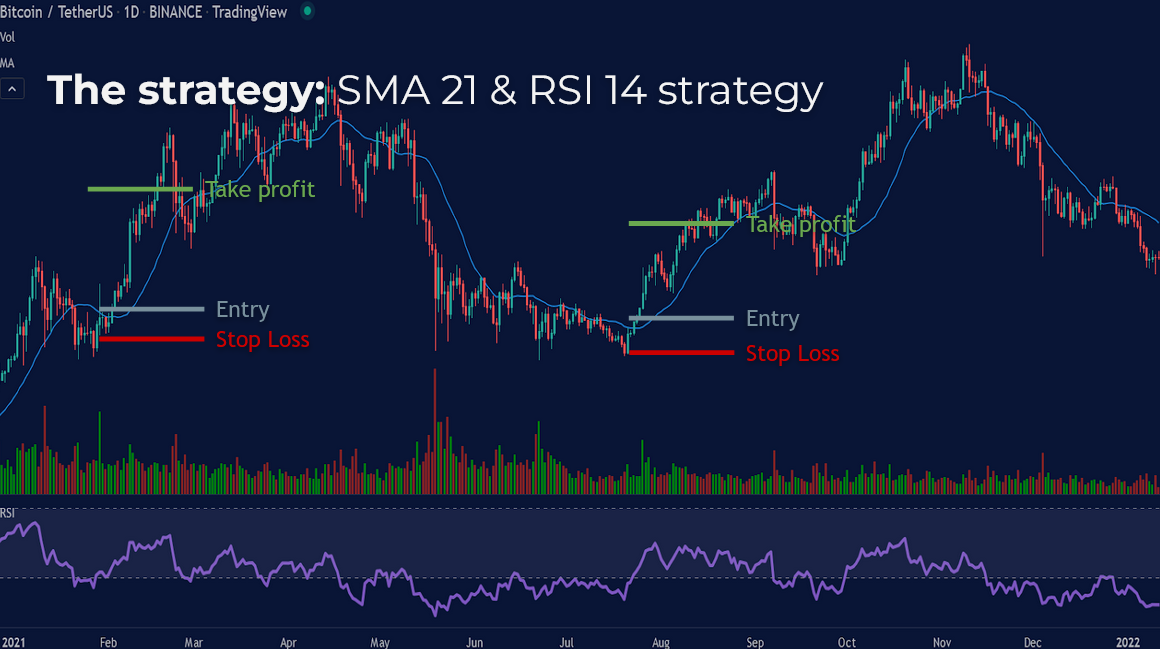### Pseudocode

In pseudocode the strategy looks like this

The question here is if we want to buy when the price crosses above 21 SMA, which is a single buy moment or buy when the close price is above 21 sma (which can be any candle above sma from that crossing point on)
The same thing goes for the RSI. Do we buy on the rsi crossover or on any candle where the rsi is above 50.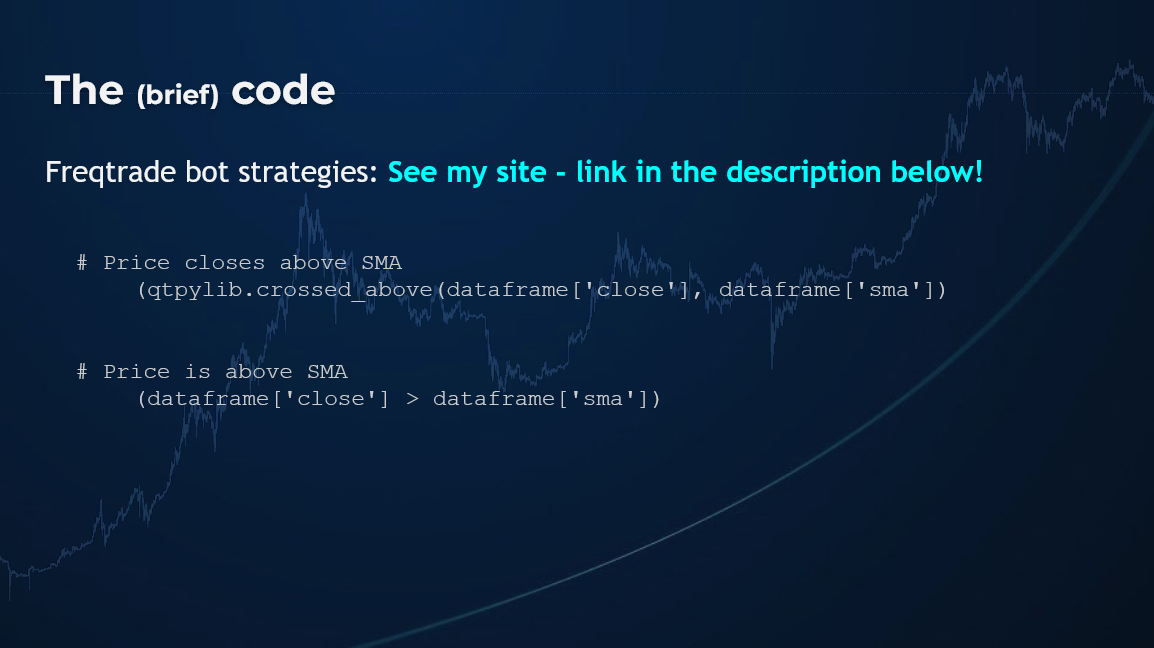The code for this bot is based on the decision to enter trades if price is crossing above sma of if price is just above the SMA altogether

And to give you an example of what I mean…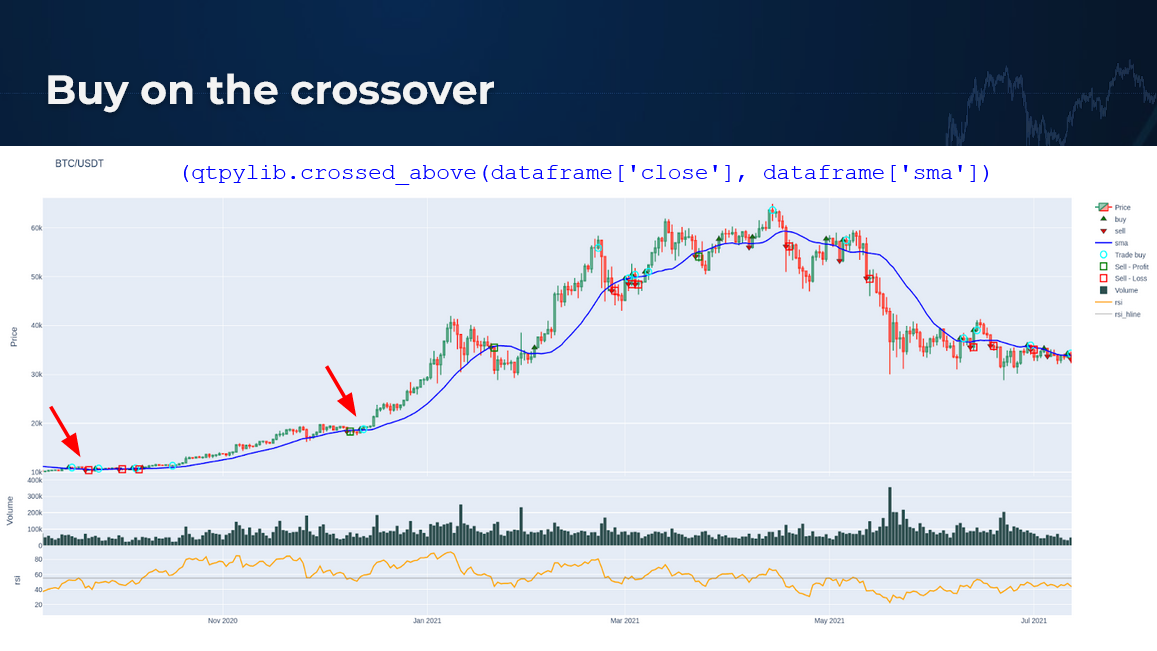Here in this plot you can see the light blue circles which are buy moments on the moment the price closes above the simple moving average
These buy moments occur one day after when the candle crosses above the SMA. these signal candles have the buy signal.

Only on these crossovers you allow the bot to enter a trade

The Pro of this approach is that there are no entry signals when the uptrend is almost ending but price is not below sma yet.
Con is that the crossover moments do not occur often, so you have to enter every signal otherwise you’ll risk to miss out on a possible large trend.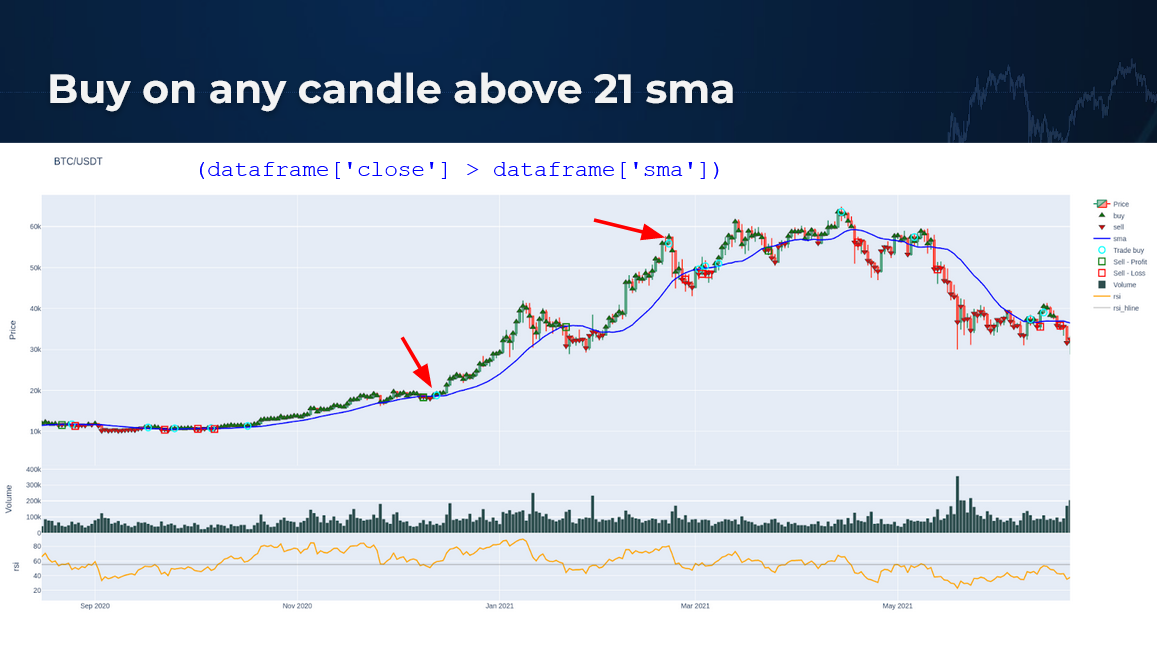This plot shows the results where you enter a trade on every moment the price is above the sma.
And you can see on the plot that every candle above the sma has a buy signal.

The benefit of this strategy is that you can still enter a trade after the initial crossover has occurred but the parabolic run has yet to come. And so you benefit from this price increase.
But the disadvantage is that you also have the possibility to enter a trade at the end of a parabolic run.
And therefore chance of a dip is higher than further increase of the price.
The bot enters a trade just before the price drops below the sma.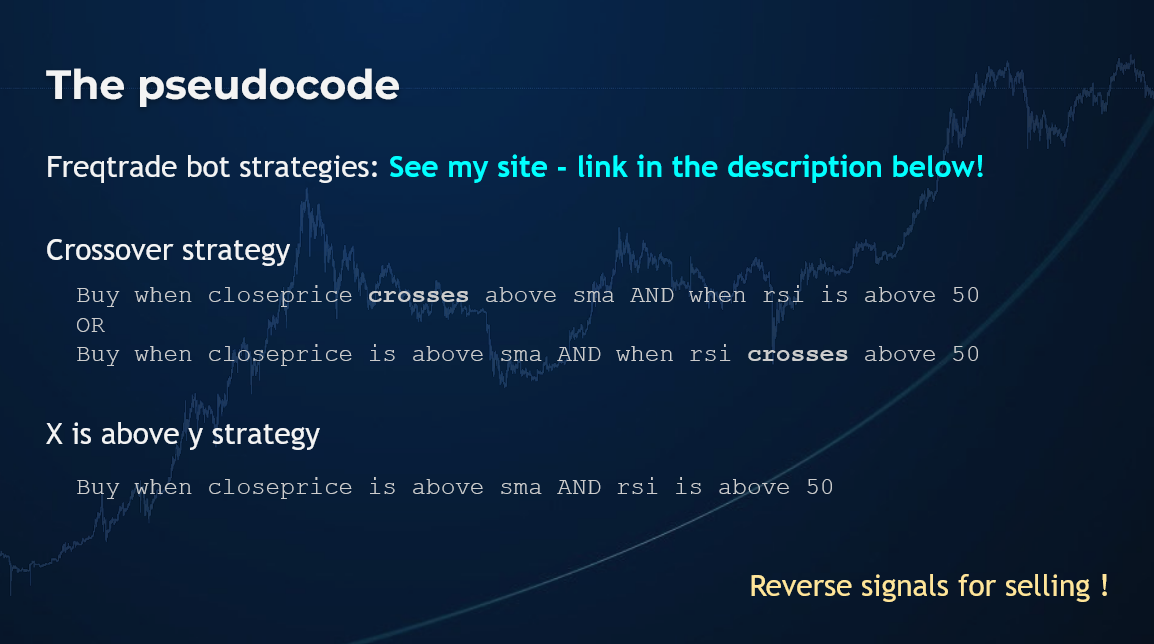So for this occasion I will test the two types of signals and see which has the best results.

Also with the use of RSI the same question goes. Do I want to enter on the crossover of on every candle where rsi is above 50.

### Initial backtest results

After backtesting and writing down all the backtest information in a sheet I have the following results: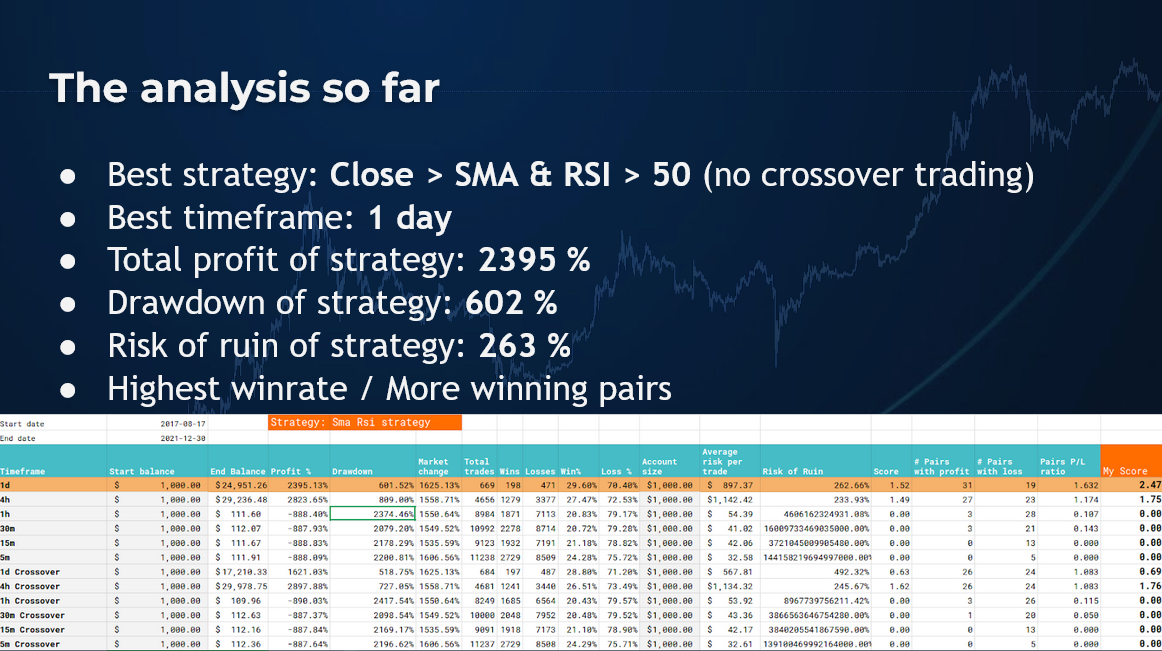The strategy where trades can be entered when close price is above sma 21 and rsi 14 is above 50 on the one day timeframe seems to give the best results.

Trading on only the crossover signals do not seem to give better results or are less risky.
Also the amount of trades between those two different trading ideas are not very much different.

My initial thought was that more trades could occur when buy signals are given when close price or rsi are just above their indicators.

Therefore also entering riskier trades at the ends of trends.
While that might still be the case it apparently does not happen often enough to downgrade the performance.
Even better, it might still catch a small run op with another coin and take profit over there as well.

The performance of the sma rsi strategy on the 4 hour timeframe where crossovers are triggering buys has a higher profit but the drawdown is also much higher and the winrate is slightly worse,
also the amount of winning pairs is not as good as the 1 day version so this timeframe wins

And thats the strategy that I am going to improve further with the help of the hyperopt function.

### Hyperparameter optimisation

As you can see the hyperopt session proposed some additional improvements.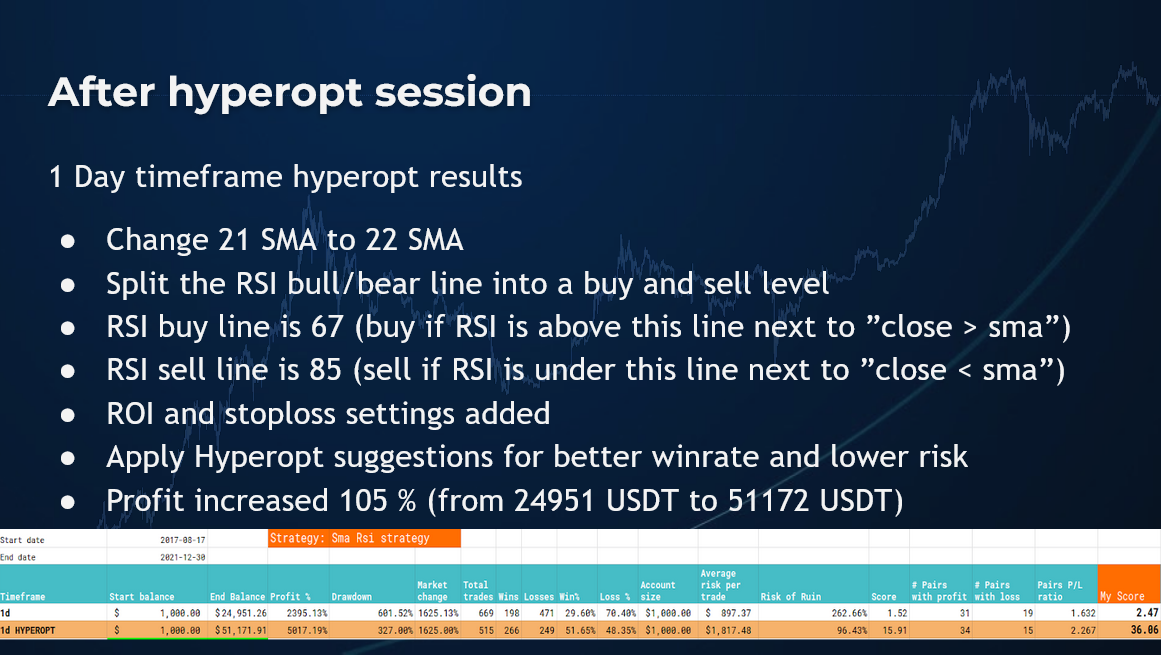Apparently changing the SMA to 22 gave better results

What I also did is splitting the initial rsi buy threshold into two levels that have to be crossed by the rsi indicator
A RSI buy level was implemented and the RSI indicator should be over that level to initiate confirmations for buying

A second sell level is used where RSI has to be below to confirm that the upward trend is stopped
If RSI is above that level there is no sell confirmation.
So this level apparently indicates a very strong trend where it is not advised to sell.

Also the Hyperopt suggested some buy levels with the roi settings and a percentage based stoploss level

This all doubled the original 2395 percent profit to 5017 percent
It ls nice to see that testing the indicator settings with hyperopt clearly produced better results than the original setting.

### Conclusions

So, again, The conclusions of my analysis of this strategy are

Best timeframe for this SMA RSI strategy is the one day timeframe

It is preferred to adjust the sma to a 22 day average,
And use a buy and sell RSI level
and also using ROI and stoploss settings proposed
THis doubled the profit of the initial strategy parameter settings to over 5000% over the backtesting period

In theory you could have potentially slightly over 51.000 dollar if you started with 1000 dollar a couple of years ago with these indicators

The winrate is 51%
And drawdown is 327%
The risk of ruin is 96%

###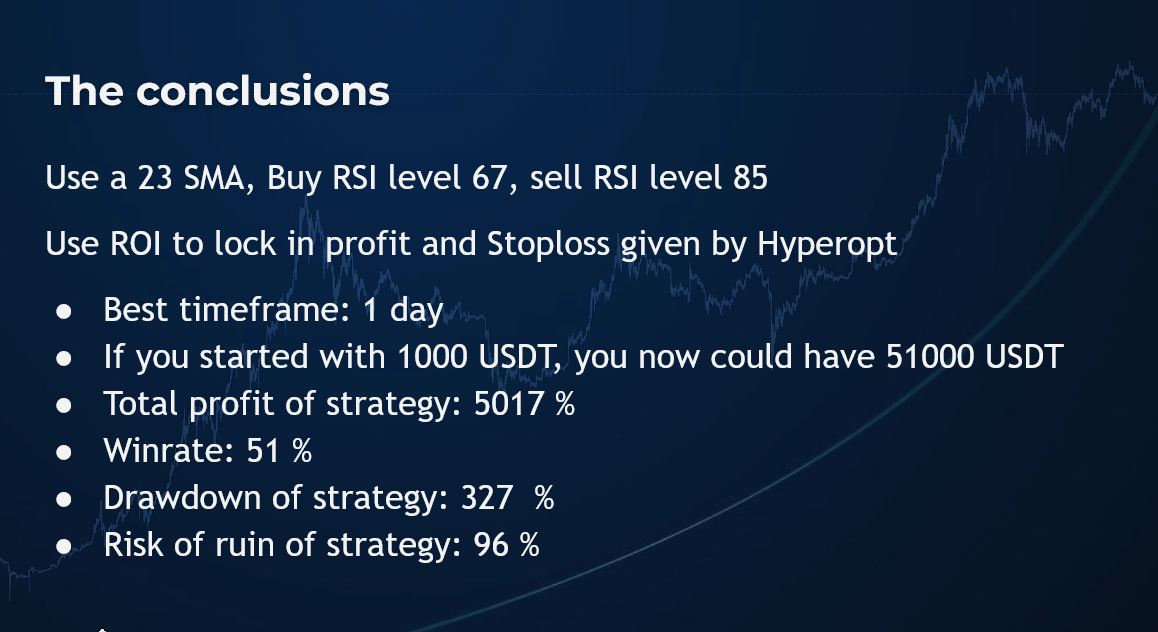Strategy League

So after I entered both results in my overall strategy comparison I get the following: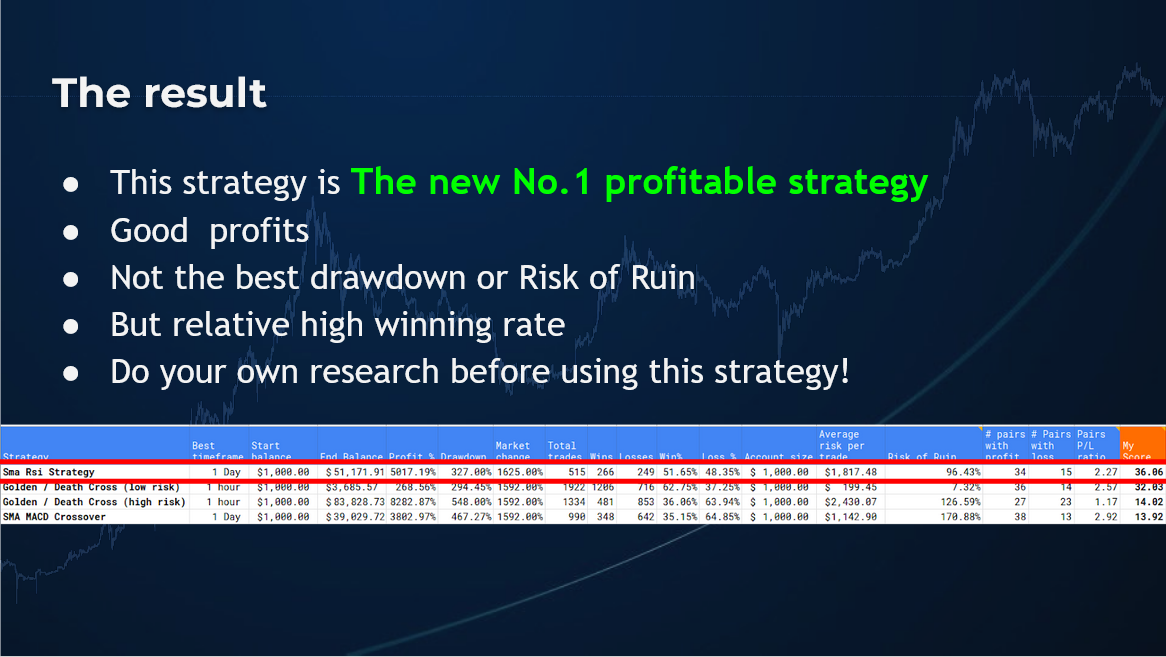The endresult is that this strategy is more profitable than the earlier tested strategies
It might have a lower winrate and higher risk of ruin than the low risk golden and death cross
But because the profits are so high in comparison this strat gets the first place

So all in all this strategy looks like it’s a profitable for trading with digital assets.

However before you decide to trade with this strategy, please do your own results and backtest according to your own methodology to be sure this strategy is for you.

### The strategy code

``````# --- Do not remove these libs ---
from pandas import DataFrame

# --- Custom libs here ---
import talib.abstract as ta

# Class should have same name as file
class SmaRsi(IStrategy):
# This strategy does not use crossovers but just enters/exits trades
# when 'is above' / 'is under' conditions are met.
timeframe = "1d"
stoploss = -100.0
minimal_roi = {"0": 100.0}

# --- Plotting ---

# Use this section if you want to plot the indicators on a chart after backtesting
plot_config = {
'main_plot': {
# Create sma line
'sma': {'color': 'blue'},
},
'subplots': {
# Create rsi subplot
"rsi": {
'rsi': {'color': 'orange'},
'rsi_hline': {'color': 'grey','plotly': {'opacity': 0.4}}
},
},
}

def populate_indicators(self, dataframe: DataFrame, metadata: dict) -> DataFrame:
dataframe["sma"] = ta.SMA(dataframe, timeperiod=21)
dataframe["rsi"] = ta.RSI(dataframe, timeperiod=14)
dataframe["rsi_hline"] = 50
return dataframe

dataframe.loc[
(
# Buy when close price > sma and rsi > rsi_hline (no crossover signal, just above...)
(dataframe['close'] > dataframe['sma'])
& (dataframe['rsi'] > dataframe['rsi_hline'])
),

return dataframe

def populate_sell_trend(self, dataframe: DataFrame, metadata: dict) -> DataFrame:
dataframe.loc[
(
# Sell when close price < sma and rsi < rsi_hline (no crossover signal, just below...)
(dataframe['close'] < dataframe['sma'])
& (dataframe['rsi'] < dataframe['rsi_hline'])
),
'sell'] = 1

return dataframe``````

### The hyperopt code

``````# --- Do not remove these libs ---
import numpy as np  # noqa
import pandas as pd  # noqa
from functools import reduce
from pandas import DataFrame
from freqtrade.strategy import (BooleanParameter, CategoricalParameter, DecimalParameter,IStrategy, IntParameter)

# --- Custom libs here ---
import talib.abstract as ta

# Class should have same name as file
class SmaRsiHopt(IStrategy):
# This strategy does not use crossovers but just enters/exits trades
# when 'is above' / 'is under' conditions are met.
timeframe = "1d"
stoploss = -100.0
minimal_roi = {"0": 100.0}

# --- Plotting ---

# Use this section if you want to plot the indicators on a chart after backtesting
plot_config = {
'main_plot': {
# Create sma line
'sma': {'color': 'blue'},
},
'subplots': {
# Create rsi subplot
"rsi": {
'rsi': {'color': 'orange'},
'rsi_sell_hline': {'color': 'grey','plotly': {'opacity': 0.4}}
},
},
}

# --- Define spaces for the indicators ---

# Buy space - UNCOMMENT THIS FOR HYPEROPTING
sma = IntParameter(13, 56, default=21, space="buy")
rsi_sell_hline = IntParameter(75, 95, default=85, space="sell")

def populate_indicators(self, dataframe: DataFrame, metadata: dict) -> DataFrame:

for val in self.sma.range:
dataframe[f'sma_{val}'] = ta.SMA(dataframe, timeperiod=val)

dataframe["rsi"] = ta.RSI(dataframe, timeperiod=14)

return dataframe

conditions = []
conditions.append(
(dataframe['close'] > dataframe[f'sma_{self.sma.value}'])
)

if conditions:
dataframe.loc[
reduce(lambda x, y: x & y, conditions),

return dataframe

def populate_sell_trend(self, dataframe: DataFrame, metadata: dict) -> DataFrame:
conditions = []
conditions.append(
(dataframe['close'] < dataframe[f'sma_{self.sma.value}'])
& (dataframe['rsi'] < self.rsi_sell_hline.value )
)

if conditions:
dataframe.loc[
reduce(lambda x, y: x & y, conditions),
'sell'] = 1

return dataframe
``````

### The hyperopt json results

``````{
"strategy_name": "SmaRsiHopt",
"params": {
"trailing": {
"trailing_stop": false,
"trailing_stop_positive": null,
"trailing_stop_positive_offset": 0.0,
"trailing_only_offset_is_reached": false
},
"sma": 22
},
"sell": {
"rsi_sell_hline": 85
},
"protection": {},
"roi": {
"0": 0.577,
"10832": 0.379,
"22671": 0.15,
"54827": 0
},
"stoploss": {
"stoploss": -0.276
}
},
"ft_stratparam_v": 1,
"export_time": "2022-01-11 16:58:24.161738+00:00"
}``````I'm just a regular Dutch dad with a passion for crypto, trading, technology and learning. This channel is my personal journey into the world of Crypto, blockchain, programming, trading bots, trading strategies, NFT's, Defi and many things more.